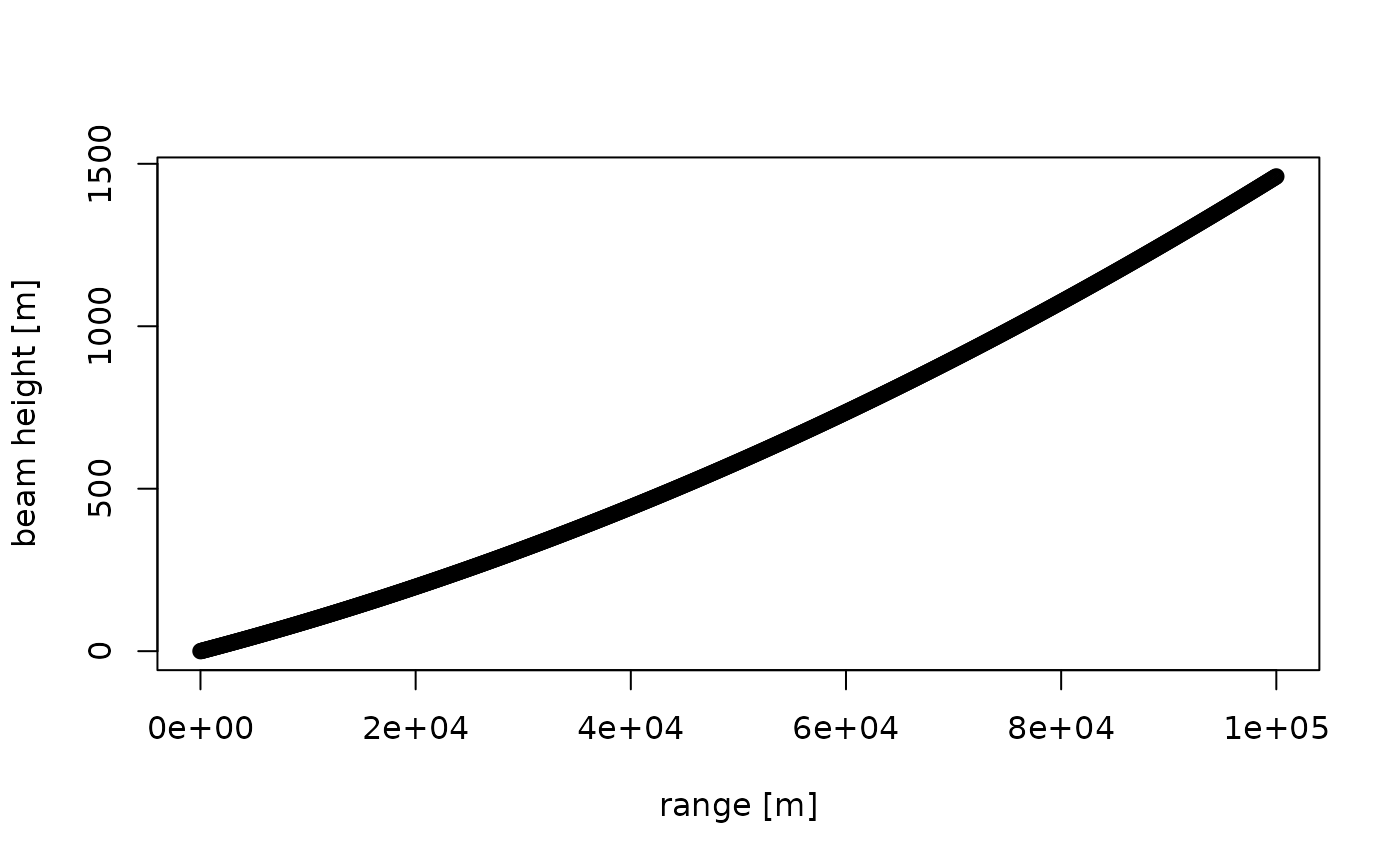Calculates the height of a radar beam as a function of elevation and range, assuming the beam is emitted at surface level.

beam_height(range, elev, k = 4/3, lat = 35, re = 6378, rp = 6357)

## Arguments

range

numeric. Slant range in m, the length of the skywave path between target and the radar antenna.

elev

numeric. Beam elevation in degrees.

k

Standard refraction coefficient.

lat

Geodetic latitude of the radar in degrees.

re

rp

## Value

numeric. Beam height in m.

## Details

To account for refraction of the beam towards the earth's surface, an effective earth's radius of k * (true radius) is assumed, with k = 4/3.

The earth's radius is approximated as a point on a spheroid surface, with re the longer equatorial radius, and rp the shorter polar radius. Typically uncertainties in refraction coefficient are relatively large, making oblateness of the earth and the dependence of earth radius with latitude only a small correction. Using default values assumes an average earth's radius of 6371 km.

## Examples

# beam height in meters at 10 km range for a 1 degree elevation beam:
beam_height(10000, 1)
#>  180.4081

# beam height in meters at 10 km range for a 3 and 5 degree elevation beam:
beam_height(10000, c(3, 5))
#>  529.229 877.398

# define ranges from 0 to 1000000 meter (100 km), in steps of 100 m:
range <- seq(0, 100000, 100)

# plot the beam height of the 0.5 degree elevation beam:
plot(range, beam_height(range, 0.5), ylab = "beam height [m]", xlab = "range [m]")